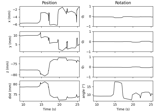# mne.viz.plot_chpi_snr#

mne.viz.plot_chpi_snr(snr_dict, axes=None)[source]#

Plot time-varying SNR estimates of the HPI coils.

Parameters:
snr_dict`dict`

The dictionary returned by `compute_chpi_snr`. Must have keys `times`, `freqs`, `TYPE_snr`, `TYPE_power`, and `TYPE_resid` (where `TYPE` can be `mag` or `grad` or both).

axes

Figure axes in which to draw the SNR, power, and residual plots. The number of axes should be 3× the number of MEG sensor types present in `snr_dict`. If `None` (the default), a new `Figure` is created with the required number of axes.

Returns:
figinstance of `matplotlib.figure.Figure`

A figure with subplots for SNR, power, and residual variance, separately for magnetometers and/or gradiometers (depending on what is present in `snr_dict`).

Notes

If you supply a list of existing `Axes`, then the figure legend will not be drawn automatically. If you still want it, running `fig.legend(loc='right', title='cHPI frequencies')` will recreate it.

New in v0.24.

## Examples using `mne.viz.plot_chpi_snr`#Extracting and visualizing subject head movement

Extracting and visualizing subject head movement